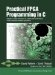# CO_PAR_BREAK

` void co_par_break(); `

co.h

#### Description

This function is used to explicitly specify each cycle boundary in a block of C code. When invoked in your C program, this function instructs the optimizer to insert a cycle boundary and suppress the generation of parallel logic.

None.

None.

#### Notes

This function is used to more precisely control the generation of parallelism in your C code. For example, if you want the optimizer to generate four clock cycles for a loop, simply add three co_par_break statements, as in the following example:

` do {   // Cycle 1 operations   co_par_break();   // Cycle 2 operations   co_par_break();   // Cycle 3 operations   co_par_break();   // Cycle 4 operations } while (...); `

If the optimizer generates more than four cycles for this block of code, that means that some operations in one of the cycles cannot be performed in parallel.Practical FPGA Programming in C
ISBN: 0131543180
EAN: 2147483647
Year: 2005
Pages: 208

Similar book on Amazon